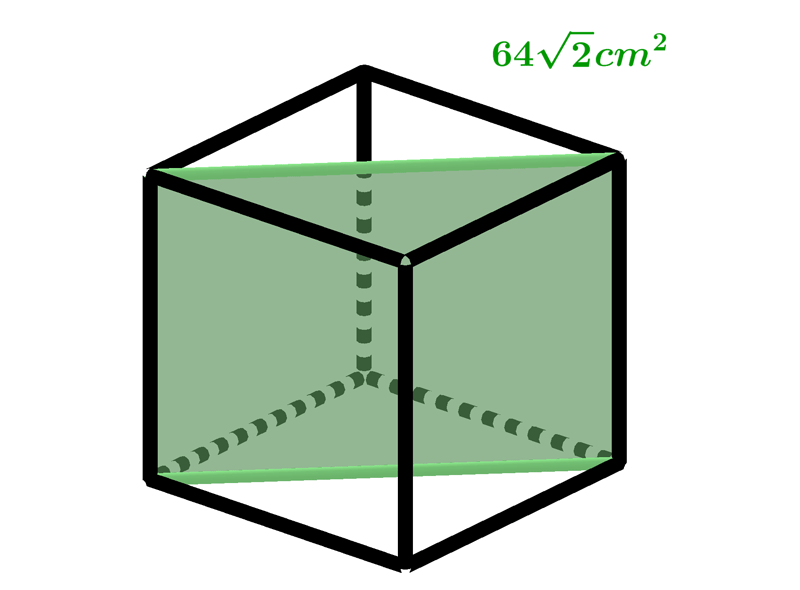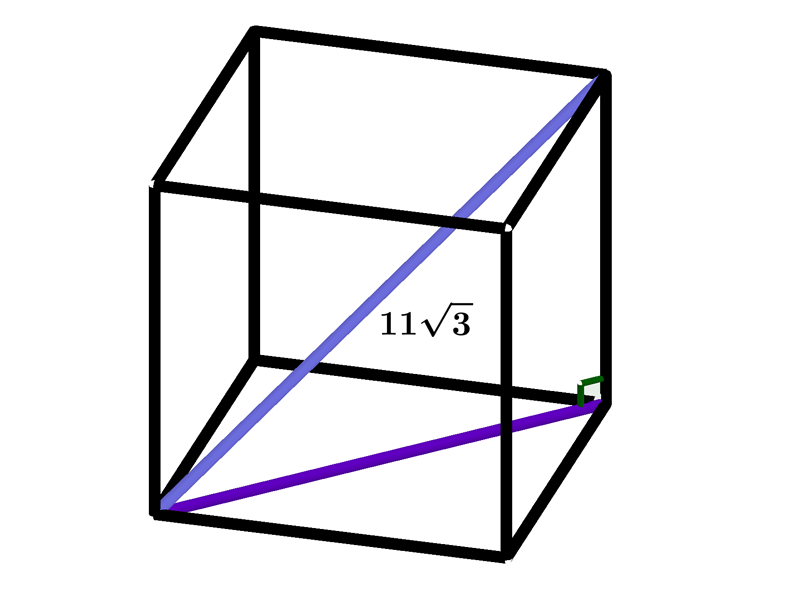Math Calculators, Lessons and Formulas

It is time to solve your math problem

mathportal.org
• Solid figures
• Cube, cuboid and Prism
• Cube and cuboid

# Cube and cuboid

ans:
syntax error
C
DEL
ANS
±
(
)
÷
×
7
8
9
4
5
6
+
1
2
3
=
0
.
auto next question
calculator
•  Question 1: 1 pts The lengths of three edges of the cuboid are $6, 8$ and $10 cm.$ Find the sum of lengths of all edges of the cuboid.
 $96cm$ $48cm$ $72cm$ $24cm$
•  Question 2: 1 pts The length of a diagonal of a cuboid is $13 cm$. Find the length of the third edge of the cuboid if two edges are $3 cm$ and $12 cm$.
 $12cm$ $8cm$ $6cm$ $4cm$
•  Question 3: 1 pts Given that the surface area of a cube is $6cm$, find the length of one of its sides.
 $3cm$ $0.5cm$ $1cm$ $2cm$
•  Question 4: 1 pts If the sum of the lengths of the edges of a cube is $48 cm$, the volume of that cube is $96 cm^{3}$
•  Question 5: 2 pts The surface area of a cube is $648 cm^{2}$, find the surface area of one of its sides.
•  Question 6: 2 pts Calculate the surface area of the cube if the surface area of the axial section of the cube is $64\sqrt{2} cm^{2}.$$169cm^{2}$ $196cm^{2}$ $296cm^{2}$ $384cm^{2}$
•  Question 7: 2 pts The diagonal of a cube is $11\sqrt{3} cm.$ Find the volume of that cube?$V=$
•  Question 8: 2 pts The volume of cuboid is $500 cm^{3}.$ Find the length of its diagonal if two of his edges has length $5 cm$ and $10 cm.$
 $15cm$ $19cm$ $25cm$ $32cm$
•  Question 9: 3 pts How many liters of water can you put in the container of the shape of a cuboid whose dimensions are $20 cm$, $60 cm$ and $5 cm$?
 $18$ liters $12$litera $6$ liters $3$ liters
•  Question 10: 3 pts The dimensions of a cuboid are in ratio $1:3:4$ and the volume is $96cm^{3}.$ Find the total surface area of the cuboid.
Total surface area$=$
•  Question 11: 3 pts Measured numbers of the surface and volume of the cube are the same. The length of the edge of the cube is $6$.
•  Question 12: 3 pts By what factor is the volume of a cube increased if each of its sides is tripled?
 $3$ times $6$ times $9$ times $27$ times